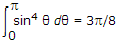Courses

# Test: Electromagnetic Field Theory - 8

## 25 Questions MCQ Test Electromagnetic Theory | Test: Electromagnetic Field Theory - 8

Description
This mock test of Test: Electromagnetic Field Theory - 8 for Electrical Engineering (EE) helps you for every Electrical Engineering (EE) entrance exam. This contains 25 Multiple Choice Questions for Electrical Engineering (EE) Test: Electromagnetic Field Theory - 8 (mcq) to study with solutions a complete question bank. The solved questions answers in this Test: Electromagnetic Field Theory - 8 quiz give you a good mix of easy questions and tough questions. Electrical Engineering (EE) students definitely take this Test: Electromagnetic Field Theory - 8 exercise for a better result in the exam. You can find other Test: Electromagnetic Field Theory - 8 extra questions, long questions & short questions for Electrical Engineering (EE) on EduRev as well by searching above.
QUESTION: 1

Solution:
QUESTION: 2

Solution:
QUESTION: 3

### Half power point directions for broadside is

Solution:
QUESTION: 4

What will happen to a bird sitting on a conductor?

Solution:
QUESTION: 5

Which one is correct option?

Solution:
QUESTION: 6

Given that, if the radiation intensity of an antenna is proportional to sin2 θ, where θ is the elevation angle, the antenna directivity will be

Solution:
QUESTION: 7

The term critical frequency denotes

Solution:
QUESTION: 8

The important postulate arising out of Maxwell's modification of Ampere's law is

Solution:
QUESTION: 9

Which of the following is not a wideband antenna?

Solution:
QUESTION: 10

The polarization phenomena in Faraday rotation

Solution:
QUESTION: 11

The portion of the atmosphere closest to ground is called ionosphere.

Solution:
QUESTION: 12

Which of the following relations is valid for a spherical surface?

Solution:
QUESTION: 13

When there is rapid attenuation of field inside the conductor, the field current are concentrated close to the surface of the conductor; this phenomenon is called

Solution:
QUESTION: 14

Which antenna is frequently used for local area transmission at UHF/VHF?

Solution:
QUESTION: 15

The work done in moving a charge Q on an equipotential line for a distance d will be

Solution:
QUESTION: 16

For frequency up to 1650 kHz, the transmitting antenna is used a

Solution:
QUESTION: 17

Solution:
QUESTION: 18

Which of the following is high frequency transmission line?

Solution:
QUESTION: 19

Which of the following are the properties of a medium?

Solution:
QUESTION: 20

In microwave communication, some times, microwave signals reach large distances by following the earth curvature. This phenomenon is called

Solution:
QUESTION: 21

An antenna is synonymous to a

Solution:
QUESTION: 22

Alternating current can induce voltage because it has magnetic field which is

Solution:
QUESTION: 23

Which of the following frequencies cannot be used for reliable beyond-the-horizon terrestrial communications without repeaters?

Solution:
QUESTION: 24

Maximum effective aperture (Ae)max of short dipole antenna is

Solution:
QUESTION: 25

Beamwidth of an antenna depends upon

Solution: